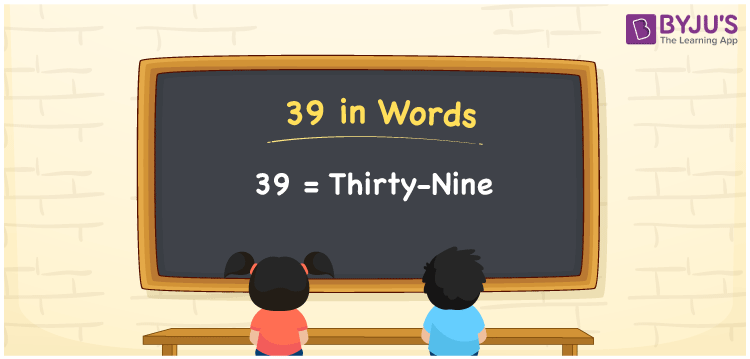# 39 in Words

We can write 39 in words as Thirty-nine. Transformation of the number 39 into words is effortless since it is a two-digit number. If you own 39 fantasy books, then you can say, “I own Thirty-nine fantasy books with me”. You can learn how to write the cardinal number 39 in words here, in this article.

 39 in words Thirty-nine Thirty-nine in Numbers 39

## 39 in English Words

Generally, we write numbers in words using the English alphabet. Thus, we can read 39 using the words in English as “Thirty-nine”.## How to Write 39 in Words?

Let us make a two-column place value chart to convert 39 to word form since it is a two-digit number, as shown below.

 Tens Ones 3 9

Here, ones = 9 and tens = 3.

This can be combined as given below.

3 × Tens + 9 × Ones

= 3 × 10 + 9 × 1

= 30 + 9

= Thirty + nine

= Thirty-nine

Therefore, 39 in words = Thirty-nine.

### Facts About the Number 39

39 is a natural number that is the successor of 38 and the predecessor of 40.

39 in words – Thirty-nine

Is 39 an even number? – No

Is 39 an odd number? – Yes

Is 39 a prime number? – No

Is 39 a composite number? – Yes

Is 39 a perfect square number? – No

Is 39 a perfect cube number? – No

How do you write 39 in Roman numerals? – XXXIX

## Frequently Asked Questions on 39 in Words

Q1

### How do you spell 39 in words?

The spelling of 39 in words is Thirty-nine, which means 39 in words can be read as Thirty-nine.
Q2

### How to write 39.75 in words?

We can write 39.75 in words as “Thirty-nine point seven five”.
Q3

### Write the value of 22 + 17 in words.

22 + 17 = 39 Thus, the value of 22 + 17, i.e. 39 in words is Thirty-nine.